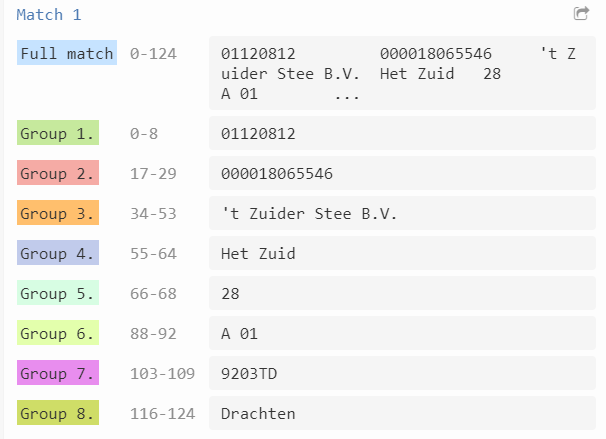Need the regex for the following input:

input string : KvK-nummer Vestigingsnr. Handelsnaam Straat Huisnummer Toevoeging Postcode Plaatsnaam

``````      01120812         000018065546     't Zuider Stee B.V.  Het Zuid   28                              9203TD       Drachten

NAW gegevens
``````

output : variable A= 01120812
variable B= 000018065546
variable c= 't Zuider Stee B.V.
vaiable D= Het Zuid
variable E= 28
variable F= empty
VariableG= 9203TD
variable H= Drachten

input pdf:

1 Like

For example the number 01120812 does its length varies? and if so does it vary in a way that it could be minimum of 8 numbers and a maximum of 10 numbers in length? for example.

to successfully write a regex that works accurately we need to know things like that.

1 Like

Have you got some more sample texts to share?

1 Like

length of columns with numbers will not vary but the alphabets size may vary and it is fixed
example- KVK number is of length - 8
Vestigingsnr.-- length is 12

This is a tough one.

Does Variable F always equal empty? If not, we need an example with something in there…

1 Like

Hello

Without any extra information a more future-proof Regex Pattern is hard to create. I have used the excessive (2 or more) spaces between each piece of text as the anchors. Variable F might need a trim in the example below or some extra brackets inserted.But regardless here is my Regex solution: regex101: build, test, and debug regex
(\d+)(\s\s+)(\d+)(?<! )(\s\s+)(.)(\s\s)(.)(\s\s+)(\d\d)(\s\s+)(\d\d\d\d\D\D)(\s\s+)(\w+)

Run your text through a “Matches” Activity using the above pattern then use the output variable in an “Assign” activity for each variable:

Variable A:

INSERTVARIABLE(0).Groups(1).ToString

Variable B:

INSERTVARIABLE(0).Groups(3).ToString

Variable C:

INSERTVARIABLE(0).Groups(5).ToString

Variable D:

INSERTVARIABLE(0).Groups(7).ToString

Variable E:

INSERTVARIABLE(0).Groups(9).ToString

Variable F:

INSERTVARIABLE(0).Groups(10).ToString

Variable G:

INSERTVARIABLE(0).Groups(11).ToString

Variable H:

INSERTVARIABLE(0).Groups(13).ToString

Have a look for yourself at the solution: regex101: build, test, and debug regex

Screenshots below

If this was the solution, please mark as solved3 Likes

Hey @Sharayu

you can also try this
arrayVar = System.Text.RegularExpressions.Regex.Split(yourStringVar,“\s{3}”)
this will return all the values and you can store them in a variable based on index

Cheers
@Sharayu

1 Like

I used the same regex, but is throwing object reference error. could you please where I have done wrong

index 5 combines the two variables output

Hello

Its not liking white space results (I think).

Try this piece of regex instead (this will ignore the white spaces):
(\d+)\s\s+(\d+)(?<! )\s\s+(.)\s\s(.)\s\s+(\d\d)\s\s+(\d\d\d\d\D\D)\s\s+(\w+)

Here is my xaml: Main.xaml (5.9 KB)
Regex101 Link: regex101: build, test, and debug regex

Let me know how you goOutput screen:

1 Like

@Sharayu

And its always good practice to check if the regex output variable is not null or empty before trying to use it, it avoids possible error. just use an if activity to check and if it is empty at least let it notify you or log that instead of breaking the entire process.

1 Like

@Sharayu

You can try replacing \s{2} instead of 3i’ve tried using this arrayVar = System.Text.RegularExpressions.Regex.Split(yourStringVar,“\s{2}”)

cheers
@Sharayu

Hello @Sharayu,

you can try this flow

please let me know if this works fine for you!
you can try @Steven_McKeering Method as well, it works as well!
Cheers
@Sharayu

3 Likes

I have a question, does the same regex works, if my input for the following field is ?

Toevoeging= “A 01” as for earlier teat data it was blank

It won’t… because there wasn’t any sample data to work with in the original postMore samples would be better next time…

Here is an new/updated piece of Regex:

** (\d+)\s\s+(\d+)(?<! )\s\s+(.)\s\s(.)\s\s+(\d\d)\s\s+(\D*\s\d*)\s\s+(\d\d\d\d\D\D)\s\s+(\w+)**

Check it out here: regex101: build, test, and debug regex

Toevoeging will now be:
INSERTVARIABLE(0).Groups(6).ToString1 Like

I get this error after changing the new input string and the regex expression. Do I need to do any other changes??

Input string- "14072124 000022720626 Stichting RADAR Randwycksingel 35 A 01 6229EG Maastricht

``````     NAW gegevens"
``````

It’s because there is only 1 space and not 2 or 3 or 4 between each like in the original post.

Using Regex.xaml (9.0 KB)
@Sharayu
give a try Cheers

1 Like